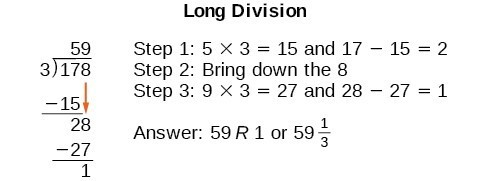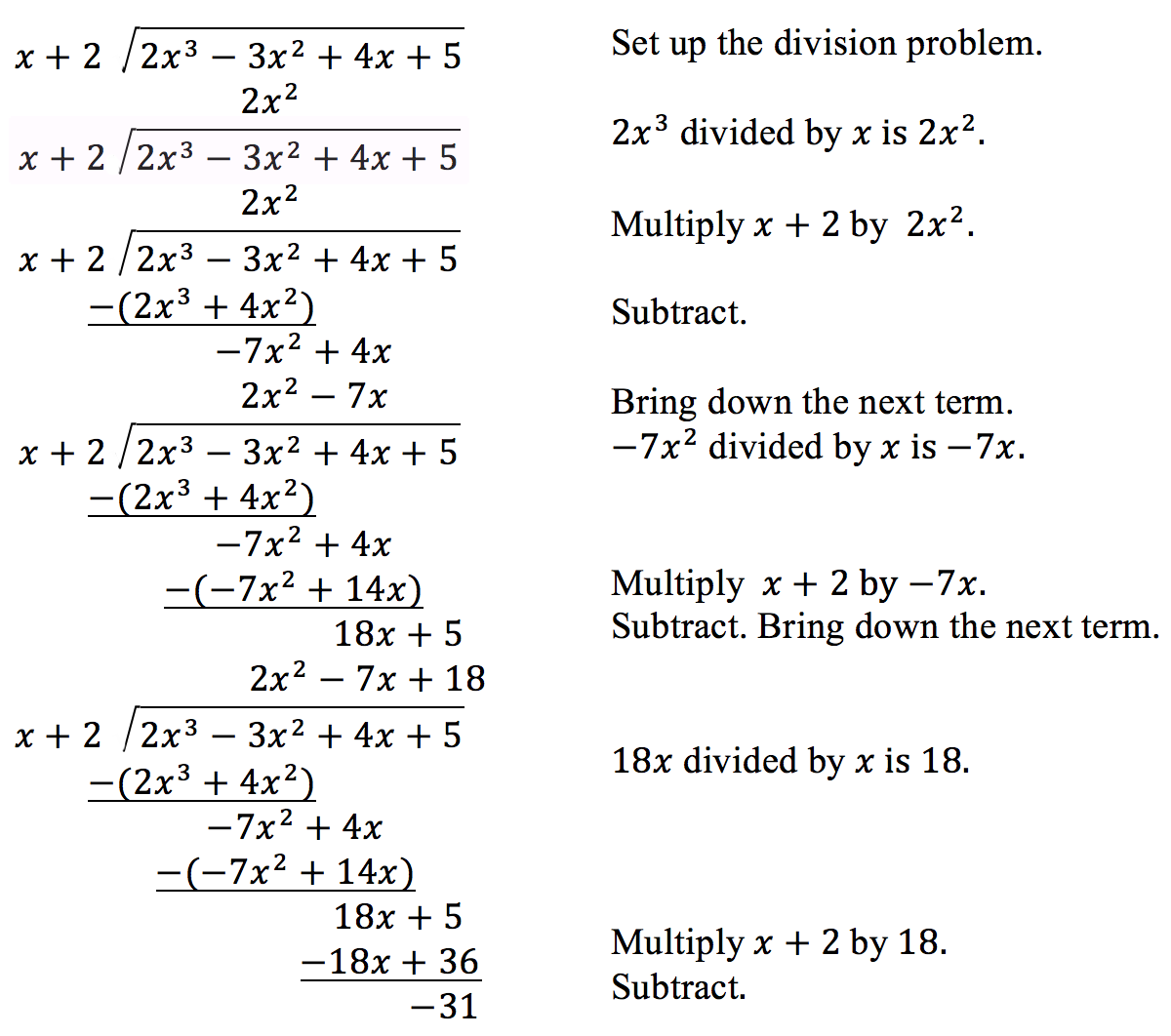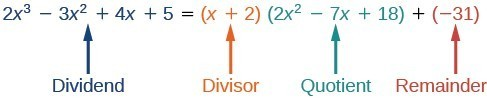## Skills Review for Partial Fractions

### Learning Outcomes

• Divide polynomials by binomials using synthetic division or long division
• Factor the greatest common factor (monomial) of a polynomial
• Factor a trinomial with a leading coefficient of 1
• Rewrite a trinomial as a four term polynomial and factor by grouping terms
• Factor difference of squares
• Factor sum or difference of cubes
• Solve rational equations by clearing denominators
• Solve systems of equations

In the Partial Fractions section, we will learn how to split fractions that have multiple terms in the denominator. This will then allow us to evaluate the resulting “split” integral. Here we will review polynomial long division, factoring polynomials, simplifying rational equations, and solving systems of equations.

## Perform Polynomial Long Division

We are familiar with the long division algorithm for ordinary arithmetic. We begin by dividing into the digits of the dividend that have the greatest place value. We divide, multiply, subtract, include the digit in the next place value position, and repeat. For example, let’s divide 178 by 3 using long division.Another way to look at the solution is as a sum of parts. This should look familiar, since it is the same method used to check division in elementary arithmetic.

$\begin{array}{l}\left(\text{divisor }\cdot \text{ quotient}\right)\text{ + remainder}\text{ = dividend}\hfill \\ \left(3\cdot 59\right)+1 = 177+1 = 178\hfill \end{array}$

We call this the Division Algorithm and will discuss it more formally after looking at an example.

Division of polynomials that contain more than one term has similarities to long division of whole numbers. We can write a polynomial dividend as the product of the divisor and the quotient added to the remainder. The terms of the polynomial division correspond to the digits (and place values) of the whole number division. This method allows us to divide two polynomials. For example, if we were to divide $2{x}^{3}-3{x}^{2}+4x+5$ by $x+2$ using the long division algorithm, it would look like this:We have found

$\frac{2{x}^{3}-3{x}^{2}+4x+5}{x+2}=2{x}^{2}-7x+18-\frac{31}{x+2}$

or

$2{x}^{3}-3{x}^{2}+4x+5=\left(x+2\right)\left(2{x}^{2}-7x+18\right)-31$

We can identify the dividenddivisorquotient, and remainder.Writing the result in this manner illustrates the Division Algorithm.

### How To: Given a polynomial and a binomial, use long division to divide the polynomial by the binomial

1. Set up the division problem.
2. Determine the first term of the quotient by dividing the leading term of the dividend by the leading term of the divisor.
3. Multiply the answer by the divisor and write it below the like terms of the dividend.
4. Subtract the bottom binomial from the terms above it.
5. Bring down the next term of the dividend.
6. Repeat steps 2–5 until reaching the last term of the dividend.
7. If the remainder is non-zero, express as a fraction using the divisor as the denominator.

### Example: Using Long Division to Divide a Second-Degree Polynomial

Divide $5{x}^{2}+3x - 2$ by $x+1$.

### Example: Using Long Division to Divide a Third-Degree Polynomial

Divide $6{x}^{3}+11{x}^{2}-31x+15$ by $3x - 2$.

## Factor Polynomials

Recall that the greatest common factor (GCF) of two numbers is the largest number that divides evenly into both numbers. For example, $4$ is the GCF of $16$ and $20$ because it is the largest number that divides evenly into both $16$ and $20$. The GCF of polynomials works the same way: $4x$ is the GCF of $16x$ and $20{x}^{2}$ because it is the largest polynomial that divides evenly into both $16x$ and $20{x}^{2}$.

Finding and factoring out a GCF from a polynomial is the first skill involved in factoring polynomials.

### Factor a GCF out of a Polynomial

When factoring a polynomial expression, our first step is to check to see if each term contains a common factor. If so, we factor out the greatest amount we can from each term. To make it less challenging to find this GCF of the polynomial terms, first look for the GCF of the coefficients, and then look for the GCF of the variables.

### A General Note: Greatest Common Factor

The greatest common factor (GCF) of a polynomial is the largest polynomial that divides evenly into each term of the polynomial.

To factor out a GCF from a polynomial, first identify the greatest common factor of the terms. You can then use the distributive property “backwards” to rewrite the polynomial in a factored form. Recall that the distributive property of multiplication over addition states that a product of a number and a sum is the same as the sum of the products.

#### Distributive Property Forward and Backward

Forward: We distribute $a$ over $b+c$.

$a\left(b+c\right)=ab+ac$.

Backward: We factor $a$ out of $ab+ac$.

$ab+ac=a\left(b+c\right)$.

We have seen that we can distribute a factor over a sum or difference. Now we see that we can “undo” the distributive property with factoring.

### Example: Factoring The Greatest Common Factor

Factor $25b^{3}+10b^{2}$.

### How To: Given a polynomial expression, factor out the greatest common factor

1. Identify the GCF of the coefficients.
2. Identify the GCF of the variables.
3. Combine to find the GCF of the expression.
4. Determine what the GCF needs to be multiplied by to obtain each term in the expression.
5. Write the factored expression as the product of the GCF and the sum of the terms we need to multiply by.

### Example: Factoring the Greatest Common Factor

Factor $6{x}^{3}{y}^{3}+45{x}^{2}{y}^{2}+21xy$.

Watch this video to see more examples of how to factor the GCF from a trinomial.

### Factor a Trinomial with Leading Coefficient 1

Although we should always begin by looking for a GCF, pulling out the GCF is not the only way that polynomial expressions can be factored. The polynomial ${x}^{2}+5x+6$ has a GCF of 1, but it can be written as the product of the factors $\left(x+2\right)$ and $\left(x+3\right)$.

Trinomials of the form ${x}^{2}+bx+c$ can be factored by finding two numbers with a product of $c$ and a sum of $b$. The trinomial ${x}^{2}+10x+16$, for example, can be factored using the numbers $2$ and $8$ because the product of these numbers is $16$ and their sum is $10$. The trinomial can be rewritten as the product of $\left(x+2\right)$ and $\left(x+8\right)$.

### A General Note: Factoring a Trinomial with Leading Coefficient 1

A trinomial of the form ${x}^{2}+bx+c$ can be written in factored form as $\left(x+p\right)\left(x+q\right)$ where $pq=c$ and $p+q=b$.

### Q & A

Can every trinomial be factored as a product of binomials?

No. Some polynomials cannot be factored. These polynomials are said to be prime.

### How To: Given a trinomial in the form ${x}^{2}+bx+c$, factor it

1. List factors of $c$.
2. Find $p$ and $q$, a pair of factors of $c$ with a sum of $b$.
3. Write the factored expression $\left(x+p\right)\left(x+q\right)$.

### Example: Factoring a Trinomial with Leading Coefficient 1

Factor ${x}^{2}+2x - 15$.

### Q & A

Does the order of the factors matter?

No. Multiplication is commutative, so the order of the factors does not matter.

### Factor by Grouping

Trinomials with leading coefficients other than 1 are slightly more complicated to factor. For these trinomials, we can factor by grouping by dividing the x term into the sum of two terms, factoring each portion of the expression separately, and then factoring out the GCF of the entire expression. The trinomial $2{x}^{2}+5x+3$ can be rewritten as $\left(2x+3\right)\left(x+1\right)$ using this process. We begin by rewriting the original expression as $2{x}^{2}+2x+3x+3$ and then factor each portion of the expression to obtain $2x\left(x+1\right)+3\left(x+1\right)$. We then pull out the GCF of $\left(x+1\right)$ to find the factored expression.

### A General Note: Factor by Grouping

To factor a trinomial of the form $a{x}^{2}+bx+c$ by grouping, we find two numbers with a product of $ac$ and a sum of $b$. We use these numbers to divide the $x$ term into the sum of two terms and factor each portion of the expression separately then factor out the GCF of the entire expression.

### How To: Given a trinomial in the form $a{x}^{2}+bx+c$, factor by grouping

1. List factors of $ac$.
2. Find $p$ and $q$, a pair of factors of $ac$ with a sum of $b$.
3. Rewrite the original expression as $a{x}^{2}+px+qx+c$.
4. Pull out the GCF of $a{x}^{2}+px$.
5. Pull out the GCF of $qx+c$.
6. Factor out the GCF of the expression.

### Example: Factoring a Trinomial by Grouping

Factor $5{x}^{2}+7x - 6$ by grouping.

### Try It

In the next video, we see another example of how to factor a trinomial by grouping.

### Factor a Difference of Squares

A difference of squares is a perfect square subtracted from a perfect square. Recall that a difference of squares can be rewritten as factors containing the same terms but opposite signs because the middle terms cancel each other out when the two factors are multiplied.

${a}^{2}-{b}^{2}=\left(a+b\right)\left(a-b\right)$

$\\$

We can use this equation to factor any differences of squares.

### A General Note: Differences of Squares

A difference of squares can be rewritten as two factors containing the same terms but opposite signs.

${a}^{2}-{b}^{2}=\left(a+b\right)\left(a-b\right)$

### How To: Given a difference of squares, factor it into binomials

1. Confirm that the first and last term are perfect squares.
2. Write the factored form as $\left(a+b\right)\left(a-b\right)$.

### Example: Factoring a Difference of Squares

Factor $9{x}^{2}-25$.

### Q & A

Is there a formula to factor the sum of squares?

No. A sum of squares cannot be factored.

Watch this video to see another example of how to factor a difference of squares.

### Factor the Sum and Difference of Cubes

Now we will look at two new special products: the sum and difference of cubes. Although the sum of squares cannot be factored, the sum of cubes can be factored into a binomial and a trinomial.

${a}^{3}+{b}^{3}=\left(a+b\right)\left({a}^{2}-ab+{b}^{2}\right)$

$\\$

Similarly, the sum of cubes can be factored into a binomial and a trinomial but with different signs.

${a}^{3}-{b}^{3}=\left(a-b\right)\left({a}^{2}+ab+{b}^{2}\right)$

$\\$

We can use the acronym SOAP to remember the signs when factoring the sum or difference of cubes. The first letter of each word relates to the signs: Same Opposite Always Positive. For example, consider the following example.

${x}^{3}-{2}^{3}=\left(x - 2\right)\left({x}^{2}+2x+4\right)$

The sign of the first 2 is the same as the sign between ${x}^{3}-{2}^{3}$. The sign of the $2x$ term is opposite the sign between ${x}^{3}-{2}^{3}$. And the sign of the last term, 4, is always positive.

### A General Note: Sum and Difference of Cubes

We can factor the sum of two cubes as

${a}^{3}+{b}^{3}=\left(a+b\right)\left({a}^{2}-ab+{b}^{2}\right)$

We can factor the difference of two cubes as

${a}^{3}-{b}^{3}=\left(a-b\right)\left({a}^{2}+ab+{b}^{2}\right)$

### How To: Given a sum of cubes or difference of cubes, factor it

1. Confirm that the first and last term are cubes, ${a}^{3}+{b}^{3}$ or ${a}^{3}-{b}^{3}$.
2. For a sum of cubes, write the factored form as $\left(a+b\right)\left({a}^{2}-ab+{b}^{2}\right)$. For a difference of cubes, write the factored form as $\left(a-b\right)\left({a}^{2}+ab+{b}^{2}\right)$.

### Example: Factoring a Sum of Cubes

Factor ${x}^{3}+512$.

### Try It

Factor the sum of cubes $216{a}^{3}+{b}^{3}$.

### Example: Factoring a Difference of Cubes

Factor $8{x}^{3}-125$.

### Try It

Factor the difference of cubes: $1,000{x}^{3}-1$.

### Try It

In the following two video examples, we show more binomials that can be factored as a sum or difference of cubes.

## Solve Rational Equations

Equations that contain rational expressions are called rational equations. For example, $\frac{2x+1}{4}=\frac{x}{3}$ is a rational equation. One of the most straightforward ways to solve a rational equation is to eliminate denominators with the common denominator and then use properties of equality to isolate the variable. This method is often used to solve linear equations that involve fractions as in the following example:

Solve  $\frac{1}{2}x-3=2-\frac{3}{4}x$ by clearing the fractions in the equation first.

Multiply both sides of the equation by $4$, the common denominator of the fractional coefficients.

$\begin{array}{c}\frac{1}{2}x-3=2-\frac{3}{4}x\\ 4\left(\frac{1}{2}x-3\right)=4\left(2-\frac{3}{4}x\right)\\\text{}\\\,\,\,\,4\left(\frac{1}{2}x\right)-4\left(3\right)=4\left(2\right)+4\left(-\frac{3}{4}x\right)\\2x-12=8-3x\\\underline{+3x}\,\,\,\,\,\,\,\,\,\,\,\,\,\,\,\,\,\,\,\,\,\,\,\underline{+3x}\\5x-12=8\,\,\,\,\,\,\,\,\\\,\,\,\,\,\,\,\underline{+12}\,\,\,\,\underline{+12} \\5x=20\\x=4\end{array}$

We could have found a common denominator and worked with fractions, but that often leads to more mistakes. We can apply the same idea to solving rational equations.  The difference between a linear equation and a rational equation is that rational equations can have polynomials in the numerator and denominator of the fractions. This means that clearing the denominator may sometimes mean multiplying the whole rational equation by a polynomial. In the next example, we will clear the denominators of a rational equation with a term that has a polynomial in the numerator.

### Example: Simplifying and Solving a Rational Equation

Solve the equation $\frac{x+5}{8}=\frac{7}{4}$.

In the next example, we show how to solve a rational equation with a binomial in the denominator of one term. We will use the common denominator to eliminate the denominators from both fractions. Note that the LCD is the product of both denominators because they do not share any common factors.

### Example: Simplifying and Solving a Rational Equation

Solve the equation $\frac{8}{x+1}=\frac{4}{3}$.

### TRY IT

Solve the equation $\dfrac{3}{x-2}=\dfrac{1}{x-1}+\dfrac{7}{(x-1)(x-2)}$.

## Solve Systems of Equations

A system of linear equations consists of two or more linear equations made up of two or more variables such that all equations in the system are considered simultaneously. To find the unique solution to a system of linear equations, we must find a numerical value for each variable in the system that will satisfy all equations in the system at the same time. Some linear systems may not have a solution and others may have an infinite number of solutions. In order for a linear system to have a unique solution, there must be at least as many equations as there are variables. Even so, this does not guarantee a unique solution.

We will first look at systems of linear equations in two variables, which consist of two equations that contain two different variables. For example, consider the following system of linear equations in two variables.

$\begin{gathered}2x+y=15\\ 3x-y=5\end{gathered}$

The solution to a system of linear equations in two variables is any ordered pair that satisfies each equation independently. In this example, the ordered pair (4, 7) is the solution to the system of linear equations. We can verify the solution by substituting the values into each equation to see if the ordered pair satisfies both equations. Shortly we will investigate methods of finding such a solution if it exists.

\begin{align}2\left(4\right)+\left(7\right)&=15&&\text{True} \\ 3\left(4\right)-\left(7\right)&=5&&\text{True} \end{align}

Two of the most common ways to solve a system of linear equations are the substitution method and the addition (elimination) method.

### How To: Given a system of two equations in two variables, solve using the substitution method.

1. Solve one of the two equations for one of the variables in terms of the other.
2. Substitute the expression for this variable into the second equation, then solve for the remaining variable.
3. Substitute that solution into either of the original equations to find the value of the first variable. If possible, write the solution as an ordered pair.
4. Check the solution in both equations.

### Example: Solving a System of Equations Using The Substitution Method

Solve the following system of equations by substitution.

$\begin{gathered}-x+y=-5 \\ 2x - 5y=1 \end{gathered}$

### How To: Given a system of equations, solve using the addition method.

1. Write both equations with x– and y-variables on the left side of the equal sign and constants on the right.
2. Write one equation above the other, lining up corresponding variables. If one of the variables in the top equation has the opposite coefficient of the same variable in the bottom equation, add the equations together, eliminating one variable. If not, use multiplication by a nonzero number so that one of the variables in the top equation has the opposite coefficient of the same variable in the bottom equation, then add the equations to eliminate the variable.
3. Solve the resulting equation for the remaining variable.
4. Substitute that value into one of the original equations and solve for the second variable.
5. Check the solution by substituting the values into the other equation.

### Example: Solving a System of Equations Using The Addition Method

Solve the given system of equations by addition.

$\begin{gathered}x+2y=-1 \\ -x+y=3 \end{gathered}$

### Example: Solving a System of Equations Using The Addition Method

Solve the given system of equations in two variables by addition.

$\begin{gathered}2x+3y=-16\\ 5x - 10y=30\end{gathered}$

### Try It

Solve the system of equations by addition.

$\begin{gathered}2x - 7y=2\\ 3x+y=-20\end{gathered}$

### How To: Given a linear system of three equations, solve for three unknowns.

1. Pick any pair of equations and solve for one variable.
2. Pick another pair of equations and solve for the same variable.
3. You have created a system of two equations in two unknowns. Solve the resulting two-by-two system.
4. Back-substitute known variables into any one of the original equations and solve for the missing variable.

### Example: Solving a System of Equations Containing Three Variables

Find a solution to the following system:

\begin{align}x - 2y+3z&=9&& \text{(1)} \\ -x+3y-z&=-6&& \text{(2)} \\ 2x - 5y+5z&=17&& \text{(3)} \end{align}

### Try It

Solve the system of equations in three variables.

\begin{align}2x+y - 2z&=-1 \\ 3x - 3y-z&=5 \\ x - 2y+3z&=6 \end{align}